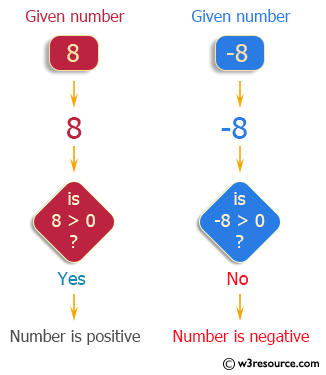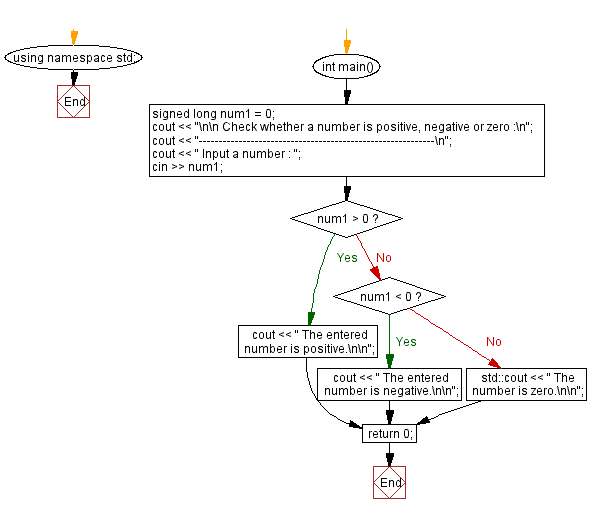﻿ C++ : Check whether a number is positive, negative or zero

# C++ Exercises: Check whether a number is positive, negative or zero

## C++ Basic: Exercise-32 with Solution

Write a program in C++ to check whether a number is positive, negative or zero.

Pictorial Presentation:Sample Solution:

C++ Code :

``````#include <iostream>
using namespace std;

int main()
{
signed long num1 = 0;
cout << "\n\n Check whether a number is positive, negative or zero :\n";
cout << "-----------------------------------------------------------\n";
cout << " Input a number : ";
cin >> num1;
if(num1 > 0)
{
cout << " The entered number is positive.\n\n";
}
else if(num1 < 0)
{
cout << " The entered number is negative.\n\n";
}
else
{
std::cout << " The number is zero.\n\n";
}
return 0;
}
``````

Sample Output:

``` Check whether a number is positive, negative or zero :
-----------------------------------------------------------
Input a number : 8
The entered number is positive.
```

Flowchart:C++ Code Editor:

What is the difficulty level of this exercise?

﻿

## C++ Programming: Tips of the Day

Why is there no std::stou?

The most pat answer would be that the C library has no corresponding "strtou", and the C++11 string functions are all just thinly veiled wrappers around the C library functions: The std::sto* functions mirror strto*, and the std::to_string functions use sprintf.

Ref: https://bit.ly/3wtz2qA

We are closing our Disqus commenting system for some maintenanace issues. You may write to us at reach[at]yahoo[dot]com or visit us at Facebook